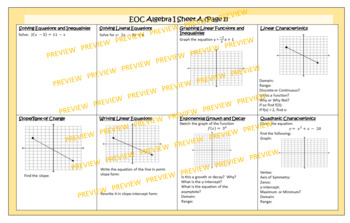Algebra 1 End of Course (EOC) Review8th - 10th, Homeschool
Subjects
Standards
Resource Type
Formats Included
• PDF
Pages
N/A

Description

Algebra 1 End of Course (EOC) Review

Do your students forget a topic when another one is introduced? You can eliminate that problem by using spiraling practice throughout the year. This 90-question resource includes everything your students need to know for your Algebra I class. Worked out answers are included for students to self-check.

This resource includes 5 sets of…

• 5 Solving Equation and Inequality Problems
• 6 Graphing Linear Functions
• 5 Slope Problems
• 3 Regression Problems
• 5 Exponential Growth and Decay
• 12 Polynomials Problems
• 5 Systems of Linear Equations
• 3 Arithmetic/Geometric Sequence
• 5 Solving Literal Equations
• 5 Linear Characteristics
• 5 Writing Linear Equations
• 5 Transformation Problems
• 11 Exponent Problems
• 4 Writing Equations from Verbal Problems
• 2 Direct Variation Problems

Here are some other Algebra I activities you may like!

Error Analysis of Multi-Step Equations using Task Cards

Solving Equations Tarsia Puzzle or Matching Activity

Polynomial Operations Escape Room using Google Forms

Regular VS Literal Equations: A Comparison Activity

Error Analysis of Multi-Step Inequalities using Task Cards

Solving One and Two-Step Inequalities: Triangle Mazes Activity

Error Analysis of Vertex and Intercept Form of Quadratics to Standard Form

Writing Slope-Intercept Form from Linear Equations, Graphs, and Tables

Error Analysis of Multiplying Polynomials

Identifying Parallel and Perpendicular Lines: \$100,000 Pyramid Activity

Error Analysis of Adding and Subtracting Polynomials

Error Analysis of Graphing Linear Inequalities

Error Analysis of Solving for y and Graphing Linear Equations

Error Analysis of Solving Equations in Slope-Intercept Form using y = mx + b to find b

Customer Tips:

How do I get TpT credit for future purchases?

Go to your "My Purchases" page. Next to each purchase, you'll see a "Provide Feedback" button. Simply click it and you will be taken to a page where you can give a quick rating and leave a short comment for the product. Each time you give feedback, TpT gives you feedback credits that you may use to lower the cost of your future purchases.

Contact me at suzy.mahoney@gmail.com with any questions!

Thank you,

Suzy Mahoney

Total Pages
N/A
Included
Teaching Duration
N/A
Report this Resource to TpT
Reported resources will be reviewed by our team. Report this resource to let us know if this resource violates TpT’s content guidelines.

Standards

to see state-specific standards (only available in the US).
Recognize situations in which a quantity grows or decays by a constant percent rate per unit interval relative to another.
Distinguish between situations that can be modeled with linear functions and with exponential functions.
Write a function that describes a relationship between two quantities.
Use the process of factoring and completing the square in a quadratic function to show zeros, extreme values, and symmetry of the graph, and interpret these in terms of a context.
Calculate and interpret the average rate of change of a function (presented symbolically or as a table) over a specified interval. Estimate the rate of change from a graph.